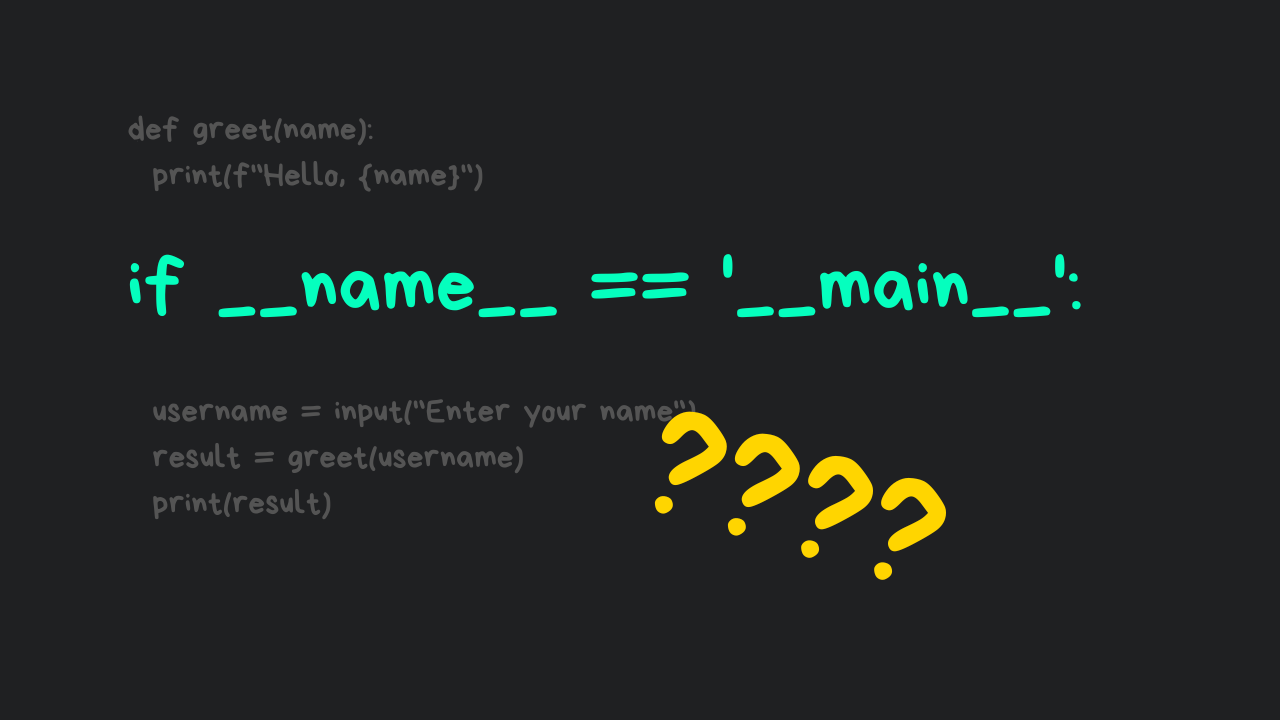## Understanding if __name__ == ‘__main__’ in Python Programs

You may have seen the `if __name__ == '__main__':` along with some code written inside this block in Python script. Have you ever wondered what this block is, and why it is used?

In this article, you’ll discover the concept of `if __name__ == '__main__'` block.

## Understanding if __name__ == ‘__main__’?

Well, `if __name__ == '__main__':` is not some magical keyword or incantation in Python rather it is a way to ensure that specific code is executed when the module is directly executed not when it is imported as a module.

What this expression implies is that only when a certain condition is met, further action should be taken. For example, if the name of the current running module (`__name__`) is the same as `"__main__"`, only the code following the `if __name__ == '__main__':` block is executed.

Here’s a Python script in which a function is defined that returns a square of the value and a print statement that prints the square of the specified integer.

If you run the script directly, it will produce the desired result, which is `4`. The issue arises when you try to reuse the `square` function in another script.

A new Python script (`square.py`) is created, and the `square` function from the previous script is imported into it.

When you run the above script, the console will display the following output.

The output shows that the code from the main script (`math_module.py`) is executed first, followed by the code from the currently running script.

You reused the `square` function, but the code inside the main script is executed automatically, which you obviously do not want. This is where you can use the `if __name__ == "__main__":` block functionality.

### Why It Is Used?

If you add the `if __name__ == "__main__":` block after the `square` function code in the main script, the code within the `if __name__ == "__main__":` block will not be executed automatically when you import the script in another module.

The `if __name__ == "__main__":` block is added to the main script (`math_module.py`), along with some code. If you import the `square` function from the main script into the second script (`square.py`), the main script’s code will no longer run automatically.

When you run the above code, you will get the following result.

You may be wondering how a single line of code can restrict the execution of code within the `if __name__ == "__main__":` block into another script.

### How Did It Happen?

Curious! How the code in the `if __name__ == "__main__":` block was not executed when you ran `square.py`.

When you run the above code, you will get the following output.

When a Python script is run, the `__name__` variable is set to `"__main__"`, indicating that the script is being run as the main program.

When a Python script is imported as a module into another script, the `__name__` variable is set to the name of the script/module.

So, code within the `if __name__ == "__main__":` block will only be executed if the script is run directly, not when it is imported as a module. When you print the name of the `square.py`, you will get the following output.

You can see that the name of the main script (`math_module.py`) changed which is why the code in the `if __name__ == "__main__":` block didn’t get executed when the `square.py` script was executed.

## Conclusion

In a nutshell, `if __name__ == "__main__":` is like a gatekeeper that ensures that a specific portion of your Python script runs only when you directly execute that script. It keeps things clean, and organized, and helps you create versatile Python modules for your projects.

The `if __name__ == "__main__":` can be very handy in larger projects where you have multiple scripts working together. You can import functions and classes from one script into another without running the whole program every time.

🏆Other articles you might be interested in if you liked this one

That’s all for now

Keep Coding✌✌